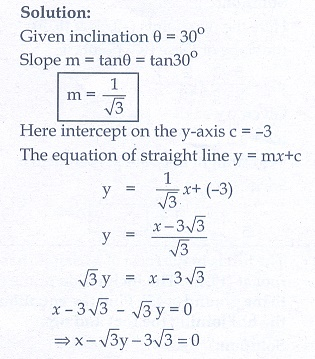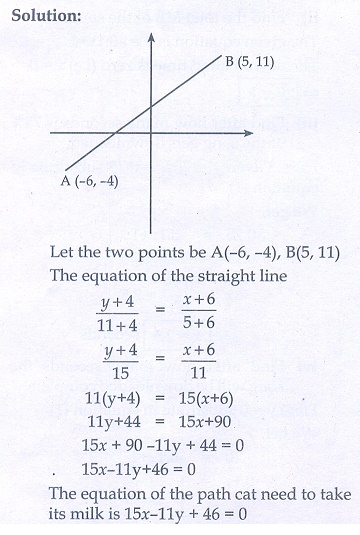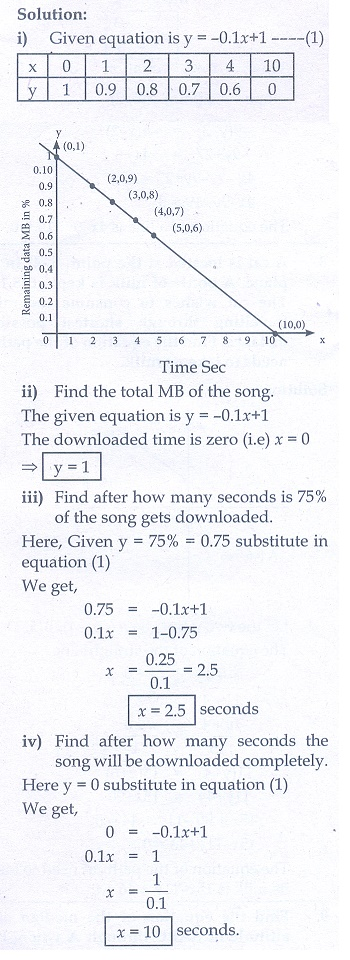Home | | Maths 10th Std | Exercise 5.3: Straight line

# Exercise 5.3: Straight line

Maths Book back answers and solution for Exercise questions - Mathematics : Coordinate Geometry: Straight line: Exercise Problem Questions with Answer

## Exercise 5.3

1. Find the equation of a straight line passing through the mid-point of a line segment joining the points (1, - 5) , (4,2) and parallel to

(i) X axis

(ii) Y axis2. The equation of a straight line is 2(x − y ) + 5 = 0 . Find its slope, inclination and intercept on the Y axis.3. Find the equation of a line whose inclination is 30˚ and making an intercept -3 on the Y axis.4. Find the slope and y intercept of √3x + (1-√3)y = 3.5. Find the value of ‘a’, if the line through (–2,3) and (8,5) is perpendicular to y = ax + 26. The hill in the form of a right triangle has its foot at (19, 3) . The inclination of the hill to the ground is 45˚. Find the equation of the hill joining the foot and top.7. Find the equation of a line through the given pair of points

(i) (2,2/3) and (-1/2,-2) (ii) (2, 3) and (- 7, -1)8. A cat is located at the point(- 6, - 4) in xy plane. A bottle of milk is kept at (5, 11) . The cat wish to consume the milk travelling through shortest possible distance. Find the equation of the path it needs to take its milk.9. Find the equation of the median and altitude of  ΔABC through A where the vertices are A(6, 2) , B(- 5, -1) and C(1, 9)10. Find the equation of a straight line which has slope -5/4 and passing through the point (–1,2).11. You are downloading a song. The percent y (in decimal form) of mega bytes remaining to get downloaded in x seconds is given by y = −0.1x + 1 .

(i) graph the equation

(ii) find the total MB of the song.

(iii) after how many seconds will 75% of the song gets downloaded?12. Find the equation of a line whose intercepts on the x and y axes are given below.

(i) 4, –6

(ii) -5, 3/413. Find the intercepts made by the following lines on the coordinate axes.

(i) 3x − 2y − 6 = 0

(ii) 4x + 3y + 12 = 014. Find the equation of a straight line

(i) passing through (1, - 4) and has intercepts which are in the ratio 2:5

(ii) passing through (-8, 4) and making equal intercepts on the coordinate axes